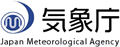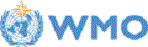Home World Climate Climate System Monitoring El Niño Monitoring NWP Model Prediction Global Warming Climate in Japan Training Module Press release Links
HOME
AAA
Description of probabilistic forecast

# Description of probabilistic forecast

## Introduction

The Japan Meteorological Agency (JMA) provides tercile probabilistic forecasts for 3-month-averaged sea surface temperature, surface temperature and precipitation over the global. The ordered probit model is used to calibrate tercile probabilistic forecasts using 30-year hindcasts (1981-2010). The thresholds of tercile are determined so that the climatological chance of occurrence for each category is 33.3 % for the hindcast period from 1981 to 2010. The specification of the numerical prediction model is described on the model outline page.

## Explanation of the ordered probit model

The ordered probit model is an extension of the binary probit model that can be used in cases where there are multiple and ranked discrete dependent variables. Considering the simple case where the dependent variable Y takes the values 0, 1 and 2 as in the binary probit model, we define an unobserved index function Y* as

```
Y* = X β + ε
```

and assume

```
Y = 0 if  Y* < k1,
Y = 1 if k1 ≤ Y* < k2,
Y = 2 if k2 ≤ Y*,
```

where k1 and k2 are "cut points" and k1 < k2. Then, the conditional probabilities Pr(Y=0 | X), Pr(Y=1 | X), and Pr (Y=2 | X) can be written as

```
Pr(Y=0 | X) = Pr(X β + ε < k1) = Pr(ε < - X β + k1) = F(- X β + k1),
Pr(Y=2 | X) = Pr(X β + ε > k2) = Pr(ε > - X β + k2) = 1 - F(- X β + k2),
Pr(Y=1 | X) = 1 - Pr(Y=0) - Pr(Y=2) = F(- X β + k2) - F(- X β + k1),
```

where F is the cumulative distribution function of residual ε. In the ordered probit model, it is assumed that the residual ε has the standard normal distribution N(0,1). Thus, F is the cumulative function of N(0,1). The statistical model is used to calculate the lower tercile (Y=0), the middle tercile (Y=1), and the upper tercile (Y=2) probabilities for 3-month-averaged model outputs. In the case of precipitation, the predictor X is the ensemble mean 1/4 power transformed precipitation to get better fit to the Gaussian distribution, otherwise the predictor X is a simple ensemble mean. In order to determine the three parameters of the statistical model, β, k1 and k2 by the maximun likelihood estimation method using the 30-year (1981-2010) hindcast data. The skill of the tailored probabilistic forecasts is cross-validated, and shown in the verification pages ( maps of BSS and ROC area scores, and reliability diagrams and ROC curves).

## Explanation of forecast maps

The probabilistic forecast maps over global or Asia region are shown. For example, Fig. 1 shows most likely Categories of surface temperature (left), precipitation (middle) and sea surface temperature (right) in the Asian region for June-July-August 2015. On the maps of surface temperature, the colors range from light to dark red is for above normal, grey is for normal and from light blue to purple is for below normal. On the maps for precipitation, the colors range from light green to blue is for above normal, grey is for normal and from dark yellow to red is for below normal. Please click any areas of your interest on the maps to display the tercile probabilities (Fig. 2). The verifications are also available from links in the forecast page at station points, where the calibrated hindcasts were verified over the 9 nearrest grids. Please note that due to a limited size of hindcast cases, the verification scores are used for guidance of forecast performance.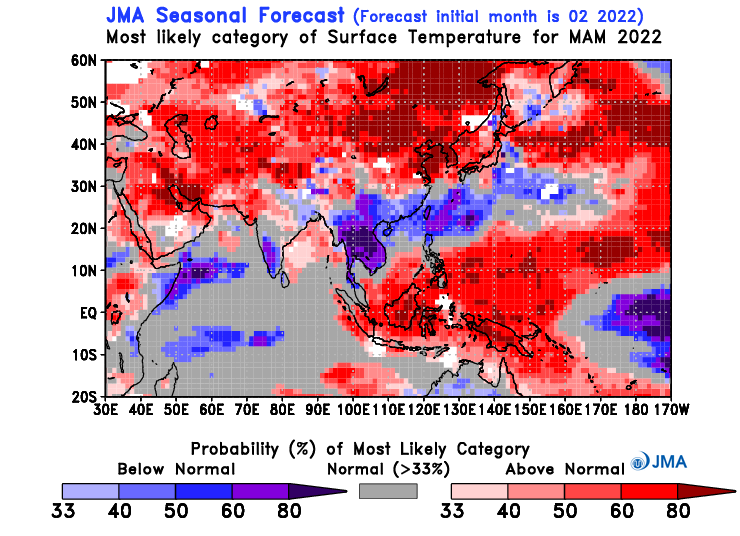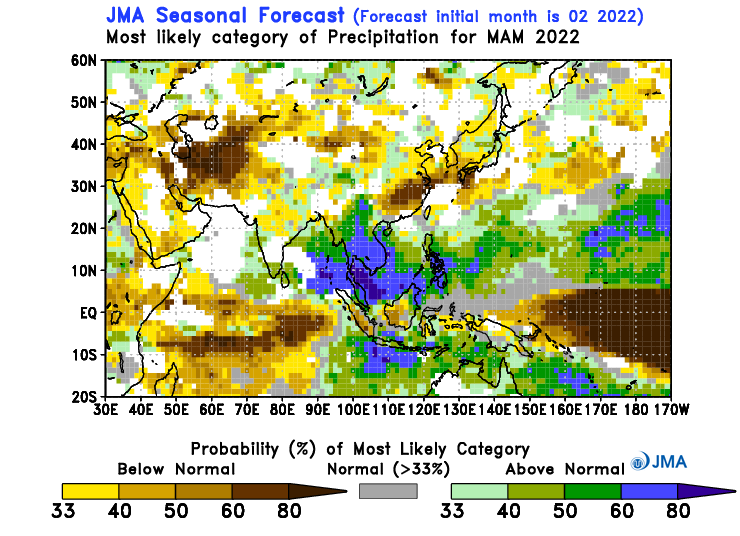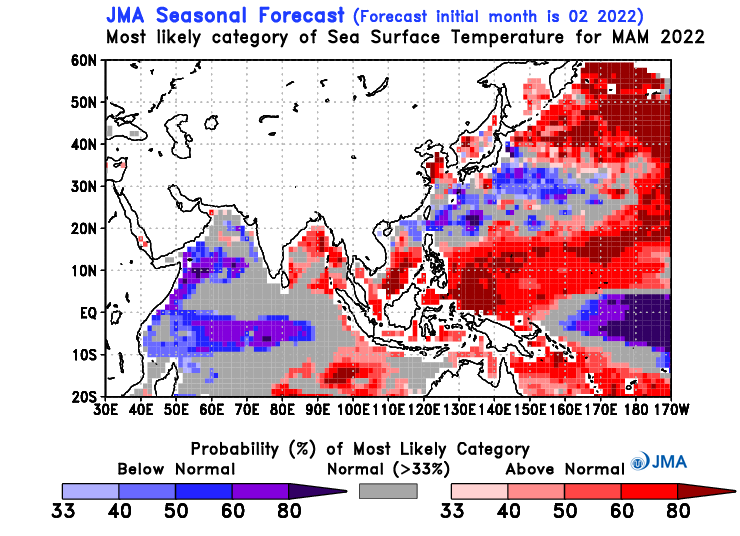Figure 1 Most likely Categories of surface temperature (left), precipitation (middle) and sea surface temperature (right) in Asia region for June-July-August 2015.Figure 2 The probability for each category in Northwestern Pacific (140E, 25N). In the following cases, scores are not plotted. insufficient prediction skill (ROC area < 0.5) skill for sea surface temperature in land grid

page top

Tokyo Climate Center, Climate Prediction Division, 1-3-4 Otemachi, Chiyoda-ku, Tokyo, Japan.
Copyright(C) 2002. Japan Meteorological Agency. - Legal Notice -